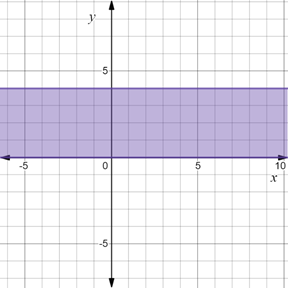# To graph : The region provided by the set { ( x , y ) | 0 ≤ y ≤ 4 } .### Precalculus: Mathematics for Calcu...

6th Edition
Stewart + 5 others
Publisher: Cengage Learning
ISBN: 9780840068071### Precalculus: Mathematics for Calcu...

6th Edition
Stewart + 5 others
Publisher: Cengage Learning
ISBN: 9780840068071

#### Solutions

Chapter 1.8, Problem 28E
To determine

## To graph: The region provided by the set {(x,y)|0≤y≤4} .

Expert Solution

### Explanation of Solution

Given information:

The set, {(x,y)|0y4} .

Graph:

The graph of the region provided by the set {(x,y)|0y4} is sketched in the coordinate plane.

Construct horizontal lines y=0 and y=4 and shaded the region between it.

The region obtained is provided below,Interpretation:

The region provided by the set {(x,y)|0y4} is shaded region. The horizontal line at y=0 and y=4 are dark lines because they are included in the set.The region consists of all those points whose y-coordinate lie between y=0 and y=4 . Therefore, shaded region is the region between horizontal lines y=0 and y=4 and these two horizontal lines are included in the region.

### Have a homework question?

Subscribe to bartleby learn! Ask subject matter experts 30 homework questions each month. Plus, you’ll have access to millions of step-by-step textbook answers!